avcontentteam — Updated On October 25th, 2023

If there is one language every data science professional should know – it is SQL. SQL stands for Structured Query Language. It is a query language used to access data from relational databases and is widely used in data science. It is especially powerful for querying datasets for data analysis. SQL can be used with various relational database systems like MySQL, Microsoft SQL Server, PostgreSQL, etc., for executing complex queries.

Positions like data analyst, data engineer, and data scientist require you to have good expertise in SQL, python programming language, and machine learning. In this article, we will be looking at questions that can test a data science professional on SQL for data analytics or data engineering. To ensure you’re well-prepared, we recommend starting with the free beginner tutorial guide for SQL. It’s essential to go through this comprehensive course before testing your skills.

This SQL skill test focuses on practical aspects and challenges people encounter while using SQL. In this article, we provide answers to these test questions, allowing you to review and identify areas for improvement

To learn SQL or to improve your SQL skills, you can have a look at these resources.

## SQL Skill Test Questions and Answers

#### Q1. Which of the following is the correct order of occurrence in a typical SQL statement?

A. select, group by, where, having
B. select, where, group by, having
C. select, where, having, group by
D. select, having, where, group by

Solution: B

Explanation: Where clause always comes before group by clause and having clause always comes after group by. The select statement always comes first.

Question Context: 2 to 12
STUDENT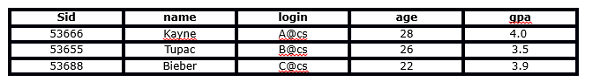ENROLLED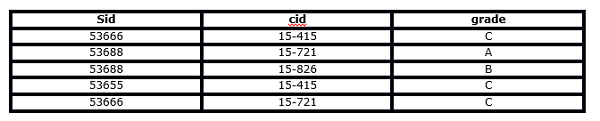#### Q2. Which of the following is the correct outcome of the SQL query below?

`Query: SELECT cid FROM ENROLLED WHERE grade = 'C'`

A. Extract the course ids(cid) where the student received the grade C in the course
B. Extract the unique course ids(cid) where the student received the grade C in the course
C. Error
D. None of these

Solution: A

Explanation: The query will extract the course ids where students receive the grade “C” in the course.

#### Q3. Which of the following is the correct outcome of the SQL query below?

`Query: SELECT DISTINCT cid FROM ENROLLED WHERE grade = 'C'`

A. Extract the course ids where the student receive the grade C in the course
B. Extract the Distinct course ids where the student receive the grade of C in the course
C. Error
D. None of these

Solution: B

Explanation: By using the DISTINCT keyword, you can extract the distinct course ids where students received a grade of C in the course.

#### Q4. Which of the following is the correct outcome of the SQL query below?

`Query: SELECT name, cid FROM student, enrolled WHERE student.sid = enrolled.sid AND enrolled.grade = 'C'`

A. Returns the name of all students and their corresponding course ids
B. Returns the name of students and their corresponding course id where they have received grade C
C. Error
D. None of these

Solution: B

Explanation: In the above query, we perform an inner join on the ENROLLED and STUDENT tables. Then, it will evaluate the WHERE condition, and then it will return the name of the students and the corresponding course id where they received the grade of C.

#### Q5. Which of the following is the correct outcome of the SQL query below?

``Query: SELECT student.name, enrolled.grade FROM student, enrolled WHERE student.sid = enrolled.sid AND enrolled.cid = '15-415' AND enrolled.grade IN ('A', 'B')``

A. Returns the name and grade of the students who took the course ’15-415′ and got a grade ’A’ or ‘B’ in that course
B. Returns the name and grade of the students who took the course ’15-415′ but didn’t get a grade ‘A’ or ‘B’ in that course
C. Error
D. None of these

Solution: A

Explanation: The above query first joined the ENROLLED and STUDENT tables. Then it will evaluate the where condition and then return the name, and grade of the students, who took 15-415 and got a grade of ‘A’ or ‘B’ in the course. But for the given two tables, it will give zero records in the output.

#### Q6. Which of the following query will find all the unique students who have taken more than one course?

`A. SELECT DISTINCT e1.sid FROM enrolled AS e1, enrolled AS e2 WHERE e1.sid != e2.sid AND e1.cid != e2.cid`
`B. SELECT DISTINCT e1.sid FROM enrolled AS e1, enrolled AS e2 WHERE e1.sid = e2.sid AND e1.cid = e2.cid`
`C. SELECT DISTINCT e1.sid FROM enrolled AS e1, enrolled AS e2 WHERE e1.sid != e2.sid AND e1.cid != e2.cid`
`D. SELECT DISTINCT e1.sid FROM enrolled AS e1, enrolled AS e2 WHERE e1.sid = e2.sid AND e1.cid != e2.cid`

Solution: D

Explanation: Option D would be the right option. This query will first apply self-join on the enrolled table, and then it evaluates the condition e1.sid = e2.sid AND e1.cid != e2.cid.

#### Q7. Which of the following statements will add a column ‘F_name’ to the STUDENT table?

`A. ALTER TABLE Student add column ( F_name varchar(20));`
`B. ALTER TABLE Student add F_name varchar(20);`
`C. ALTER TABLE Student add (F_name varchar(20));`
`D. ALTER TABLE Student add column (F_name);`

Solution: B

Explanation: ALTER TABLE command allows a user to add a new column to a table. Option B is the correct syntax of ALTER to add a column in the table.

#### Q8. Which of the following query(s) will result in a successful insertion of a record in the STUDENT table?

`Query1: INSERT INTO student (sid, name, login, age, gpa) VALUES (53888, ‘Drake’, ‘drake@cs’, 29, 3.5)`
`Query2: INSERT INTO student VALUES (53888, ‘Drake’, ‘drake@cs’, 29, 3.5)`

A. Both queries will insert the record successfully
B. Query 1 will insert the record successfully, and Query 2 will not
C. Query 2 will insert the record successfully, and Query 1 will not
D. Both queries will not be able to insert the record successfully

Solution: A

Explanation: Both queries will successfully insert a row in the student table. Query 1 is useful when you want to provide the target table, columns, and values for new tuples, and Query 2 is a Short-hand version of the insert command

#### Q9. Considering the scenario below, which of the following option(s) will insert a row in the ENROLLED table successfully?

‘Sid’ in the ENROLLED table is ‘Foreign Key’ referenced by ‘Sid’ in the STUDENT table. Now you want to insert a record into the ENROLLED table.

1. `INSERT INTO ENROLLED values(53667, '15-420', 'C');`
2. `INSERT INTO ENROLLED values(53666, '15-421', 'C');`
3. `INSERT INTO ENROLLED values(53667, '15-415', 'C');`
4. `INSERT INTO ENROLLED values(53666, '15-415', 'C');`

A. 1 and 3
B. Only 3
C. 2 and 4
D. Only 4

Solution: C

Explanation: Options 2 and 4 will run successfully because in the ENROLLED table’s ‘Sid’ column, you can insert those values which are present in STUDENT’s table ‘Sid’ columns due to the foreign key constraint.

#### Q10. Consider the following queries:

`Query1: select name from enrolled LEFT OUTER JOIN student on student.sid = enrolled.sid;`
` Query2: select name from student LEFT OUTER JOIN enrolled on student.sid = enrolled.sid;`
Which of the following option is correct?

A. Queries 1 and 2 will give the same results
B. Queries 1 and 2 will give different results
C. Query 1 will produce an error, and Query 2 will run successfully
D. Query 2 will produce an error, and Query 1 will run successfully

Solution: A

Explanation: In (LEFT JOIN, RIGHT JOIN, or FULL JOIN) OUTER SQL joins, order matters. But both queries will give the same results because both are dependent on the records present in the table and which column is selected.

#### Q11. Which of the following statements will modify the data type of the “Sid” column in the ENROLLED table?

Note: There is no foreign key relationship between tables STUDENT and ENROLLED.

`A. ALTER TABLE ENROLLED MODIFY (sid varchar(100));`
`B. ALTER TABLE ENROLLED MODIFY sid varchar(100);`
`C. ALTER TABLE ENROLLED MODIFY column (sid varchar(100));`
`D. ALTER TABLE ENROLLED MODIFY attribute (sid varchar(100));`

Solution: A

Explanation: The “ALTER TABLE MODIFY” is used to modify column definitions in a table. So option A is correct.

#### Q12. Which of the following statement will remove the ‘Sid’ column from the ENROLLED table?

Note: There is no foreign key relationship between tables STUDENT and ENROLLED.

`A. ALTER TABLE ENROLLED DROP (sid varchar(10) );`
`B. ALTER TABLE ENROLLED DROP COLUMN (sid varchar(10) );`
`C. ALTER TABLE ENROLLED DROP COLUMN Sid;`
`D. ALTER TABLE ENROLLED MODIFY (sid);`

Solution: C

Explanation: The “ALTER TABLE DROP COLUMN” can be used to drop a column from the table. So Option C is the right answer.

#### Q13. Which of the following command(s) is/are related to transaction control in SQL?

A. ROLLBACK
B. COMMIT
C. SAVEPOINT
D. All of the above

Solution: D

Explanation: All are related to transaction control in SQL.

#### Q14. Which of the following is true for a primary key?

A. It can take a value more than once
B. It can take null values
C. It can’t take null values
D. None of these

Solution: C

Explanation: In a relational schema, there exists only one primary key, and it can’t take null values. So option C is the correct answer.

#### Q15. What is the difference between a primary key and a unique key?

A. Primary key cannot be a date variable, whereas a unique key can be
B. You can have only one primary key, whereas you can have multiple unique keys
C. Primary key can take null values, but the unique key cannot be null values
D. None of these

Solution: B

Explanation: You can create a date variable as a primary key in a table. In a relational schema, you can have only one primary key and there may be multiple unique keys present in the table. A unique key can take null values.

#### Q16. Which of the following statement(s) is/are true for UPDATE in SQL?

1. You can update only a single table using the UPDATE command
2. You can update multiple tables using the UPDATE command
3. In the UPDATE command, you must list what columns to update with their new values (separated by commas).
4. To update multiple targeted records, you need to specify the UPDATE command using the WHERE clause.

Select the correct option:

A. 1, 3 and 4
B. 2, 3 and 4
C. 3 and 4
D. 1 only

Solution: A

Explanation: Options are self-explanatory.

#### Q17. Which of the following is true for TRUNCATE in SQL?

A. It is usually slower than the DELETE command
B. It is usually faster than the DELETE command
C. There is no comparison between DELETE & TRUNCATE
D. Truncate command can be rolled back
E. None of these

Solution: B

Explanation: TRUNCATE is faster than delete bcoz truncate is a DDL command. So it does not produce any rollback information, and the storage space is released while the delete command is a DML command. Also, it produces rollback information, and space is not deallocated using the delete command.

#### Q18. Which of the following statement is correct about the ‘CREATE TABLE’ command while creating a table?

A. We need to assign a datatype to each column
B. We have flexibility in SQL. We can assign a data type to a column even after creating a table
C. It is mandatory to insert at least a single row while creating a table
D. None of these

Solution: A

Explanation: Each column must possess behavioral attributes like data types and precision to build the structure of the table.

#### Q19. Which of the following are the synonyms for the ‘column’ and ‘row’ of a table?

1. Row = [Tuple, Record]
2. Column = [Field, Attribute]
3. Row = [Tuple, Attribute]
4. Columns = [Field, Record]

Select the correct option:

A. 1 and 2
B. 3 and 4
C. Only 1
D. Only 2

Solution: A

Explanation: In DBMS records are also known as tuples and rows. And columns are known as attributes and fields.

#### Q20. Which of the following operator is used for comparing ‘NULL’ values in SQL?

A. Equal
B. IS
C. IN
D. None of Above

Solution: B

Explanation: In SQL, if you want to compare a null value you need to use IS statement.

#### Q21. Which of the following statement(s) is/are true about the “HAVING” and “WHERE” clause in SQL?

1. “WHERE” is always used before “GROUP BY” and HAVING after “GROUP BY”
2. “WHERE” is always used after “GROUP BY” and “HAVING” before “GROUP BY”
3. “WHERE” is used to filter rows but “HAVING” is used to filter groups
4. “WHERE” is used to filter groups but “HAVING” is used to filter rows

Select the correct option:

A. 1 and 3
B. 1 and 4
C. 2 and 3
D. 2 and 4

Solution: A

Explanation: HAVING is performed after GROUP BY. If you have to apply some conditions to get results. you need to use WHERE before group by. Aggregate functions are used with the group by.

#### Q22. Identify which of the following column “A” or “C” given in the below table is a “Primary Key” or “Foreign Key”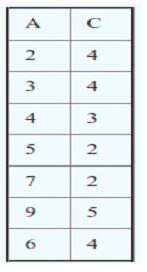Note: We have defined ‘Foreign Key’ and ‘Primary Key’ in a single table

A. Column ‘A’ is Foreign Key, and Column ‘C’ is Primary Key’
B. Column ‘C’ is Foreign Key, and Column ‘A’ is ‘Primary Key’
C. Both can be ‘Primary Key’
D. Based on the above table, we cannot tell which column is ‘Primary Key’ and which is ‘Foreign Key’

Solution: B

Explanation: Column A is taking unique values, and column A doesn’t have null values. So it can be considered the Primary key of this table. Whereas B is the example of a foreign key because all values present in this column are already present in column A.

#### Q23. What are the tuples additionally deleted to preserve reference integrity when the rows (2,4) are deleted from the below table?

Suppose you are using ‘ON DELETE CASCADE’.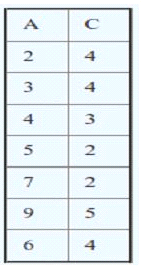Note: We have defined ‘Foreign Key’ and ‘Primary Key’ in a single table

A. (5,2), (7,2), (9,5)
B. (5,2), (7,2)
C. (5,2), (7,2), (9,5), (3,4)
D. (5,2), (7,2), (9,5),(6,4)

Solution: A

Explanation: When (2,4) is deleted. Since C is a foreign key referring to A with delete on cascade, all entries with value 2 in C must be deleted. So (5, 2) and (7, 2) are deleted. As a result of this, 5 and 7 are deleted from A, which causes (9, 5) to be deleted.

#### Q24. What will be the output for the following queries given the below scenario?

You are given a table/relation, “EMPLOYEE,” which has two columns (‘Name’ and ‘Salary’). The Salary column in this table has some NULL values. Now, I want to find out the records which have null values.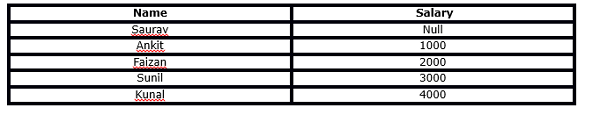`Query 1. SELECT * FROM EMPLOYEE WHERE Salary <> NULL;`
`Query 2. SELECT * FROM EMPLOYEE WHERE Salary = NULL;`

A. Query 1 will give the last 4 rows as output (excluding null value)
B. Query 2 will give the first row as output (only record containing null value)
C. Query 1 and Query 2 both will give the same result
D. Can’t say

Solution: C

Explanation: If we compare(<>, =) Salary, it will give 0 records. Below are the following reasons:

1. Salary = Null is unknown
2. Salary <> Null is unknown

#### Q25. What is the difference between TRUNCATE, DELETE, and DROP? Which of the following statement(s) is/ are correct?

1. DELETE operations can be rolled back, but TRUNCATE and DROP operations cannot be rolled back.
2. DELETE operations cannot be rolled back, but TRUNCATE and DROP operations can be rolled back.
3. DELETE is an example of DML (Data Manipulation Language), but the remaining are examples of DDL (Data Definition Language).
4. All are an example of DDL

Select the correct option:

A. 1 and 3
B. 2 and 3
C. 1 and 4
D. 2 and 4
E. None of the above

Solution: A

Explanation: Options are self-explanatory.

#### Q26. Which of the following statements is correct for the output of each query given below?

Tables A and B have three columns (namely: ‘id’, ‘age’, and ‘name’). These tables have no ‘null’ values, and there are 100 records in each table.
Here are two queries based on these two tables, ‘A’ and ‘B’:

• `Query1: SELECT A.id FROM A WHERE A.age > ALL (SELECT B.age FROM B WHERE B.name = 'Ankit')`
• `Query2: SELECT A.id FROM A WHERE A.age > ANY (SELECT B.age FROM B WHERE B.name = 'Ankit')`

A. The number of tuples in the output of Query 1 will be more than or equal to the output of Query 2
B. The number of tuples in the output of Query 1 will be equal to the output of Query 2
C. The number of tuples in the output of Query 1 will be less than or equal to the output of Query 2
D. Can’t say

Solution: C

Explanation: ANY and ALL operate on subqueries that return multiple values. ANY returns true if any of the subquery values meet the condition. But in the case of ALL, it will return the records if all conditions are true. So option C is correct.

#### Q27. What is true about the relation (table) in different normal forms (1NF, 2NF, 3NF)?

1. If a relation satisfies the conditions of 1NF. It will automatically satisfy the conditions of 2NF
2. If a relation satisfies the conditions of 2NF. It will automatically satisfy the conditions of 1NF
3. If a relation satisfies the conditions of 3NF. It will automatically satisfy the conditions of 1NF
4. If a relation satisfies the conditions of 2NF. It will automatically satisfy the conditions of 3NF

Select the correct option:

A. 1 and 2
B. 2 and 3
C. 1 and 3
D. 2 and 4

Solution: B

Explanation: If a relation satisfies higher normal forms, it automatically satisfies lower normal forms also. For example, if a relation is satisfying kNF it will automatically satisfy gNF where g<=k.

#### Q28. Suppose you want to compare three keys (‘Primary Key’, ‘Super Key’, and ‘Candidate Key’) in a database. Which of the following option(s) is/are correct?

1. Minimal super key is a candidate key
2. Only one Candidate Key can be Primary Key
3. All super keys can be a candidate key
4. We cannot find the “Primary Key” from “Candidate Key”

Select the correct option:

A. 1 and 2
B. 2 and 3
C. 1 and 3
D. 2 and 4
E. 1, 2 and 3

Solution: A

Explanation: Options are self-explanatory

#### Which of the following will be the output of DA+?Note: For any X, X+ is the closure of X.

A) DA
B) DAE
C) ABCD
D) ABCDEF

Solution: B

Explanation: (DA)+ = DAE

#### Q30. What is the output of the following SQL query?

Suppose you have a table “Loan_Records”.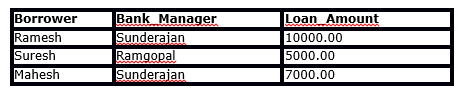`SELECT Count(*)  FROM  ( (SELECT Borrower, Bank_Manager FROM   Loan_Records) AS S NATURAL JOIN (SELECT Bank_Manager, Loan_Amount FROM   Loan_Records) AS T );`

A. 4
B. 5
C. 8
D. 10

Solution: B

Explanation: Temporary table S is given below:

Temporary table T is given below

If you apply natural join on both tables (S and T) and evaluate the condition on ‘Bank_Manager’. You will get the following intermediate table after applying natural join

“Sunderjan” appears two times in the Bank_Manager column, so there will be four entries with Bank_Manager as “Sunderjan”. So count(*) will give the 5 output in the outer query.

#### Q31. Is the “SELECT” operation in SQL equivalent to the “PROJECT” operation in relational algebra?

A. Yes, both are equivalent in all the cases
B. No, both are not equivalent

Solution: B

Explanation: In relational algebra ‘PROJECT’ operation gives the unique record, but in the case of the ‘SELECT’ operation in SQL, you need to use a distinct keyword for get unique records.

Table: AV1

Questions 32-36 are based on the above table.

#### Q32. What will be the output of the following query?

`Query 1: Select name from AV1 where name like '%a%'`

1. Saurav, Ankit, Kunal, Deepak, Swati, Faizan
2. Saurav, Kunal, Deepak, Swati, Faizan
3. Kunal, Deepak, Swati, Faizan
4. None of above

#### Q33. What will be the output for the below query?

`Query: SELECT Name from AV1 where Name LIKE '%______%';`
Note: The above operation contains 6 underscores (‘_’) used with the LIKE operator.

A. It will return names where the number of characters in names is greater than or equal to 6
B. It will return names where the number of characters in names is greater than 6
C. It will return names where the number of characters in names is less than or equal to 6
D. It will give an error

Solution: A

Explanation: The query will search for records in column ‘Name’ where the number of characters in names is greater than or equal to 6.

#### Q34. What will be the output of the below query?

`Query: SELECT Company, AVG(Salary) FROM AV1 HAVING AVG(Salary) > 1200 GROUP BY Company WHERE Salary > 1000 ;`

A.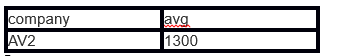B.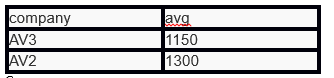C.

D. None of these

Solution: D

Explanation: This query will give the error because ‘WHERE’ is always evaluated before ‘GROUP BY’ and ‘Having’ is always evaluated after ‘GROUP BY’

#### Q35. What will be the output for the below Query 1 and Query 2?

`Query 1: SELECT MAX(Salary) FROM AV1 WHERE Salary < (SELECT MAX(Salary) from AV1);`
`Query 2: WITH S AS (SELECT Salary, ROW_NUMBER() OVER(ORDER BY Salary DESC) AS RowNum FROM AV1) SELECT Salary FROM S WHERE RowNum = 2;`

A. Query 1 output = 1200 and Query 2 output =1200
B. Query 1 output = 1200 and Query 2 output =1400
C. Query 1 output = 1400 and Query 2 output =1200
D. Query 1 output = 1400 and Query 2 output =1400

Solution: A

Explanation: Both queries will generate the second-highest salary in AV1, which is 1200. Hence A is the right option.

#### Q36. Consider the following relational schema.

`Students(rollno: integer, sname: string)`
`Courses (courseno: integer, cname: string)`
`Registration (rollno: integer, courseno: integer, percent: real)`
Now, which of the following query would be able to find the unique names of all students having scores of more than 90% in the course no 107?

A. SELECT DISTINCT S.sname FROM Students as S, Registration as R WHERE R.rollno=S.rollno AND R.courseno=107 AND R.percent >90
B. SELECT UNIQUE S.sname FROM Students as S, Registration as R WHERE R.rollno=S.rollno AND R.courseno=107 AND R.percent >90
C. SELECT sname FROM Students as S, Registration as R WHERE R.rollno=S.rollno AND R.courseno=107 AND R.percent >90
D. None of these

Solution: A

Explanation: Option A is true, Option B will give the error (“UNIQUE” is not used in SQL), and in option C, unique names will not be the output.

#### Q37. Which of the following options is when related to the following queries?

Consider the relation T1 (A, B) in which (A, B) is the primary key and the relation T2 (A, C) where A is the primary key. Assume there are no null values and no foreign keys or integrity constraints.
`Query 1: select A from T1 where A in (select A from T2)`
`Query 2: select A from T2 where A in (select A from T1)`

A. Both queries will definitely give the same result
B. Both queries may give the same result
C. Both queries will definitely give a different result
D. None of these

Solution: B

Explanation: For the same values (values should be unique) for column A in tables T1 and T2. Query 1 and Query 2 will give the same output. Hence B is true

#### Q38. Which of the following options is correct for the following queries?

`Query 1. SELECT emp.id, department.id FROM emp NATURAL JOIN department`
`Query 2. SELECT emp.id, department.id FROM department NATURAL JOIN emp`

A. Both queries will give the same outputs
B. Both queries will give different output
C. Need table structure
D. None of these

Solution: A

Explanation: For Natural joins, the order doesn’t matter. The queries will return the same results.

#### Q39. Which of the following sequences CANNOT be the sequence for the numbers examined for the below scenario?

Indexing is useful in a database for fast searching. Generally, B-tree is used for both clustered index and non-clustered index in a database. Now, you want to use Binary Search Tree instead of B-tree.
Suppose there are numbers between 1 and 100, and you want to search for the number 35 using the Binary Search Tree algorithm.

A. 10, 75, 64, 43, 60, 57, 55
B. 90, 12, 68, 34, 62, 45, 55
C. 9, 85, 47, 68, 43, 57, 55
D. 79, 14, 72, 56, 16, 53, 55

Solution: C

Explanation: In BST, on the right side of the parent number should be greater than it, but in C after 47, 43 appears that is wrong.

#### Q40. If an index scan is replaced by a sequential scan in SQL, then what will happen?

Note: The number of observations is equal to 1 million.

A. Execution will be faster
B. Execution will be slower
C. Execution will not be affected
D. None of these

Solution: B

Explanation: The addition of the index made the query execution faster since the sequential scan was replaced by the index scan.

#### Q41. Which query will take less time to execute in the below scenario?

Suppose you have a CSV file that has 3 columns (‘User_ID’, ‘Gender’, ‘Product_ID’) and 7,150,884 rows. You have created a table “train” from this file in SQL.
Now, you run Query 1 as given below and get the following output:

`Query 1: EXPLAIN select * from train where Product_ID = 'P00370853';`
OUTPUT:
`QUERY PLAN`
`--------------------------------------------------------------`
`Seq Scan on train (cost=0.00..79723.88 rows=16428 width=68)`
`Filter: ((product_id)::text = 'P00370853'::text)`
`(2 rows)`

You have now created Product_ID column as an index in train table using the below SQL query:
CREATE INDEX product_ID ON train(Product_ID)
And you run Query 2 (same as Query 1) on “train” and get the following output.

`Query 2: EXPLAIN select * from train where Product_ID = 'P00370853';`
OUTPUT:
`QUERY PLAN`
`-------------------------------------------------------------------------------`
`Bitmap Heap Scan on train (cost=829.53..40738.85 rows=35754 width=68)`
`Recheck Cond: ((product_id)::text = 'P00370853'::text)`
`-> Bitmap Index Scan on product_id (cost=0.00..820.59 rows=35754 width=0)`
`Index Cond: ((product_id)::text = 'P00370853'::text)`
`(4 rows)`

A. Query 1
B. Query 2
C. Both queries will take the same time
D. Can’t say

Solution: B

Explanation: For Query Plan for Query 1, the execution time is 79723.88, and for Query Plan for Query 2, the execution time is 40738.85. So Query 2 is taking less time.

#### Q42. Which query will take less time to execute?

Suppose you have a CSV file that has 3 columns (‘User_ID’, ‘Gender’, ‘product_ID’) and 7150884 rows. You have created a table “train” from this file in SQL.
Now, you run Query 1 (mentioned below):

`Query1: EXPLAIN SELECT * from train WHERE product_ID like '%7085%';`
Then, you created product_ID columns as an index in ‘train’ table using below SQL query:
`CREATE INDEX product_ID ON train(Product_ID)`
Suppose you run Query 2 (same as Query 1) on train table.
`Query 2: EXPLAIN SELECT * from train WHERE product_ID like '%7085%';`
Let T1 and T2 be the time taken by Query 1 and Query 2, respectively.

A. T1>T2
B. T2>T1
C. T1~T2
D. Can’t say

Solution: C

Explanation: The addition of the index didn’t change the query execution plan since the index doesn’t help for the ‘LIKE’ query.

#### Q43. Which of the following is true for the query time?

Suppose you have a table ‘Employee’. In the Employee table, you have a column named Salary. Now, you applied Query 1 on the Employee table.
`Query 1: SELECT * FROM Employee where Salary*100 > 5000;`
After that, you create an index on Salary columns, and then you re-run Query 2 (same as Query 1).
`Query 2:  SELECT * FROM Employee where Salary*100 > 5000;`
Here, Query 1 is taking T1 time, and Query 2 is taking T2 time.

A. T1 > T2
B. T2 > T1
C. T1 ~ T2
D. Can’t say

Solution: C

Explanation: The addition of the index didn’t change the query execution plan. The index on the rating will not work for the query (Salary * 100 > 5000). Theoretically, it might work in this case, but obviously, the system is not “smart” enough to work that way; But you can create an index on (Salary * 100) which will help.

#### Q44. What will be the output for the below query?

Suppose you are given a table ‘words’. The table has 2 columns – ‘id’ and ‘word’.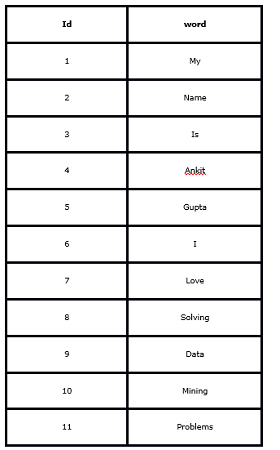Query:  select c1, c2, c3 from ( select id,  lag(word) over (order by id) as c1, word as c2, lead(word) over (order by id) as c3 from words ) as t where c2 = ‘Mining’ or c2 = ‘Problems’;

A.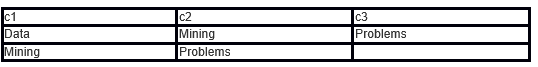B. Error

C.

D. None of these

Solution: A

#### Q45. What is true for ‘View’ in SQL?

1. It can help in providing security
2. It can be used to hide complexity
3. If there is more than one table involved in the view, we cannot perform (Data Manipulation Language) DML queries
4. When you drop the base table, the view becomes inactive.

Select the correct option:

A. 1 and 3

B. 2 and 4

C. 1, 3 and 4

D. All of these

Solution: D

Explanation: All options are correct.

#### Q46. Which of the following will be the output of Query 5 below?

Suppose I created a table called ‘avian’ using below SQL query:

Query :` CREATE TABLE avian ( emp_id SERIAL PRIMARY KEY, name varchar);`
Now, I want to insert some records in the table avian:
`Query1: INSERT INTO avian (name) VALUES(`‘FRAZY`');`
`Query2: INSERT INTO avian (name) VALUES(`‘ANKIT`');`
`Query3: INSERT INTO avian (name) VALUES('SUNIL');`
`Query4: INSERT INTO avian (name) VALUES('SAURAV');`
`Query5: Select * FROM avian;`

A.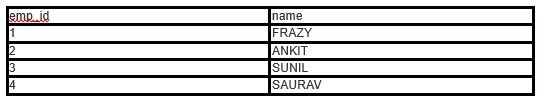B.

C. Error

D. None of these

Solution: A

Explanation: At the time of table creation Avian, we have used SERIAL for ’emp_id’, which autoincrement emp_id whenever you insert a record in table avian. Hence A is true.

We conducted a skill test to test our community on SQL and it gave 2017 a rocking start. A total of 1,666 participants registered for the skill test. Below is the distribution of scores; this will help you evaluate your performance:

You can assess your performance here. More than 700 people participated in the skill test and the highest score was 41. Here are a few statistics about the distribution.

Overall distribution

Mean Score: 22.32
Median Score: 25
Mode Score: 27

This is an interesting distribution. I think we are seeing 3 different profiles of people here:

• There are ~20 people who did not score at all. They either faced some technical problem or did not like the test, or did not know SQL.
• There is another population that looks to have normal distribution between scores 1 to 10. These people either started the competition late and hence could not get enough time, or they are just beginners in SQL.
• The third population looks to have a distribution between scores 10 and 41 and looks like a representative of people in the industry. For this group, the mean is 25.8, and the standard deviation is ~ 6.5. So anyone with a score of more than 32 is in the top 16% of the population.

## Conclusion

I hope you enjoyed taking the test and found the solutions helpful. Keep in mind that some topics like normalization, stored procedures, case statements, etc., weren’t covered in this test, as it primarily focused on conceptual knowledge of SQL. If you encountered challenges with these SQL interview questions, consider enrolling in a comprehensive SQL course to bolster your skills. There are many valuable tutorials available on platforms like YouTube to further enhance your proficiency. Happy learning!## 20 thoughts on "SQL Skill Test | SQL Quiz to Test a Data Science Professional (Updated 2023)"###### RK says:January 09, 2017 at 7:14 am
Hi in Q11: You have given Ans: A 1) option A and D are same 2) Answer should be option B? Reply###### RK says:January 09, 2017 at 7:18 am###### Benny says:January 09, 2017 at 8:14 am
Hi Ankit, Thanks for the sols. Q29 : didnt understand the qn. could you explain the qn and sol breifly. Q38 : Query2 will throw an error since emp is not an alias for employee. Solution ans is wrong Q39: For BST, i believe the series should be ordered. Can you breifly explain the sol again. Regards, B Reply###### Ankit Gupta says:January 09, 2017 at 8:49 am
Hi Benny, Thanks for noticing it. For question number 29 refer the links: 1. https://www.youtube.com/watch?v=IUPTC65B9qE 2. http://www.edugrabs.com/closure-of-a-set-x/ In question number 39, query is updated as per the skill test. If you consider the nodes after 47 in option C, we take the right sub-tree for 68. The right sub-tree can contain only those nodes which are larger than 47. But 43 is less than 47 ( because 68 is inserted at the right side of 47 and then 43 will be inserted at left side of 68, 43 suppose to be left side of 47 but it is right side of 47) Reply###### Philip says:January 09, 2017 at 5:18 pm
Can you increase the time of test at least for 4 hours . I really wanted to attempt the quiz but hardly I got 10 mins to solve , because I logged in late. They are many more things coming up. As this test are on weekend if you can increase the hour span then it will be good. Thanks Reply###### cxchan2 says:January 09, 2017 at 5:29 pm
Hi Ankit, Good job on the sql skill test. It was more like a sql refresher. I am not sure why there was no option of going back to correct or review a question. If we would have had that option, it would have been more helpful. Reply###### Jack Ma says:January 09, 2017 at 9:06 pm
This question is not related to directly related to this question. Is it possible to add the print button to the page? A lot of articles are great. But when I try to print it out. The format is terrible and print friendly. Reply###### Ankit Gupta says:January 10, 2017 at 5:24 am
Hi Philip, Feedback taken. Best! Ankit Gupta Reply###### Ankit Gupta says:January 10, 2017 at 5:24 am
Hi Philip, Feedback taken. Best! Ankit Gupta Reply###### Ankit Gupta says:January 10, 2017 at 5:26 am
Hi Jack, There are many extensions available for browsers which will do this job. Best! Ankit Gupta Reply###### Hunaidkhan Pathan says:January 10, 2017 at 2:43 pm
Hi Jack, You can use Google chrome browser and right click on any of the page you want and click on print -> it does have good options to change the print view. Also google chrome allows to save the page as PDF . Reply###### Jack Ma says:January 10, 2017 at 2:49 pm
Thank you. I do find one extension called print friendly&pdf. It is much better. Reply###### Jack Ma says:January 10, 2017 at 2:52 pm
Hi Hunaidkhan, I am using chrome. But the original print is not that good for some article, there has some overlap. But i use the extension now. it helps me a lot. Reply###### Pushpita says:January 11, 2017 at 3:22 am
Very Informative. Loved the questions and really good explanation... Reply###### Ankit Gupta says:January 11, 2017 at 5:23 am###### Ankit Sabinkar says:January 11, 2017 at 10:59 am
This was my first Hackathon and I really enjoy it. Lot more to come in future. AV Team is doing an amazing job. Thank you !!! Reply###### Ankit Gupta says:January 11, 2017 at 11:28 am